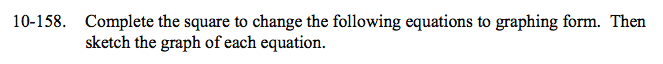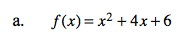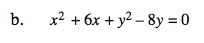Home > CCA2 > Chapter 10 > Lesson 10.3.1 > Problem10-158

10-158.
1. Complete the square to change the following equations to graphing form. Then sketch the graph of each equation. Homework Help ✎

1. f(x) = x2 + 4x + 6

2. x2 + 6x + y2 − 8y = 0f(x) − 2 = x2 + 4x + 6 − 2

Now simplify the right side of the equation and factor.You will need to complete the square twice, once for x and once for y.

(x2 + 6x + ?) + (y2 − 8y + ?) = 0 + ? + ?

will allow you to simplify the equation to

(x + ?)2 + (y − ?)2 = ?

Don't forget to graph each equation!